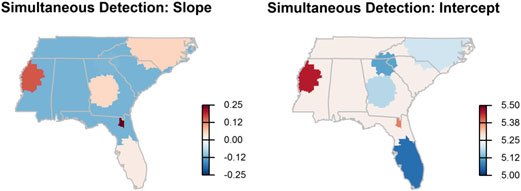# Cluster detection of spatial regression coefficients### Abstract

Popular approaches to spatial cluster detection, such as the spatial scan statistic, are defined in terms of the responses. Here, we consider a varying-coefficient regression and spatial clusters in the regression coefficients. For varying-coefficient regression, such as the geographically weighted regression, different regression coefficients are obtained for different spatial units. It is often of interest to the practitioners to identify clusters of spatial units with distinct patterns in a regression coefficient, but there is no formal statistical methodology for that. Rather, cluster identification is often ad-hoc such as by eyeballing the map of fitted regression coefficients and discerning patterns. In this paper, we develop new methodology for spatial cluster detection in the regression setting based on hypotheses testing. We evaluate our methods in terms of power and coverages for true clusters via simulation studies. For illustration, our methodology is applied to a cancer mortality dataset.

Type
Publication
Statistics in Medicine